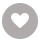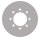# R Data Structures and Algorithms电子书

52人正在读 | 0人评论9.8

• 读书简介
• 目录
• 累计评论(0条)
• 读书简介
• 目录
• 累计评论(0条)

R Data Structures and Algorithms

R Data Structures and Algorithms

Credits

Acknowledgments

www.PacktPub.com

Why subscribe?

Preface

What this book covers

What you need for this book

Who this book is for

Conventions

Customer support

Errata

Piracy

Questions

1. Getting Started

Introduction to data structure

Abstract data type and data structure

Relationship between problem and algorithm

Basics of R

Installation of R

Basic data types in R

Operations in R

Control structures in R

If condition

If...else condition

Ifelse function

For() loop

Nested for( ) loop

While loop

Special statements in loops

Break statement

Next statement

Repeat loop

First class functions in R

Exercises

Summary

2. Algorithm Analysis

Getting started with data structure

Memory management in R

System runtime in R

Best, worst, and average cases

Computer versus algorithm

Algorithm asymptotic analysis

Upper bounds or Big O notation

Lower bounds or Big Omega notation (Ω)

Big θ notation

Simplifying rules

Classifying rules

Computation evaluation of a program

Component 1 - Assignment operator

Component 2 - Simple loop

Component 3 - Complex loop

Component 4 - Loops with conditional statements

Component 5 - Recursive statements

Analyzing problems

Space bounds

Exercises

Summary

Data types in R

Vector and atomic vector

Element data types

Factor

Matrix

Array

Dataframes

List

Object-oriented programming using R

Array-based list

Analysis of list operations

Exercises

Summary

4. Stacks and Queues

Stacks

Array-based stacks

Comparison of array-based and linked stacks

Implementing recursion

Queues

Array-based queues

Comparison of array-based and linked queues

Dictionaries

Exercises

Summary

5. Sorting Algorithms

Sorting terminology and notation

Three Θ(n²) sorting algorithms

Insertion sort

Bubble sort

Selection sort

The cost of exchange sorting

Shell sort

Merge sort

Quick sort

Heap sort

An empirical comparison of sorting algorithms

Lower bounds for sorting

Exercises

Summary

6. Exploring Search Options

Searching unsorted and sorted vectors

Self-organizing lists

Heuristic 1 - Count

Heuristic 2 - Move-to-front

Heuristic 3 - Transpose

Hashing

Hash functions

Open hashing

Closed hashing

Bucket hashing

Linear probing

Analysis of closed hashing

Deletion

Exercises

Summary

7. Indexing

Linear indexing

ISAM

Tree-based indexing

2-3 trees

B-trees

B+ trees

B-tree analysis

Exercises

Summary

8. Graphs

Terminology and representations

Graph implementations

Graph traversals

Depth-first search

Topological sort

Shortest path problems

Single-source shortest paths

Minimum-cost spanning tree

Prim's algorithm

Kruskal's algorithm

Exercises

Summary

9. Programming and Randomized Algorithms

Dynamic programming

The knapsack problem

All pairs shortest paths

Randomized algorithms

Randomized algorithms for finding large values

Skip lists

Probabilistic analysis of skip lists

Exercises

Summary

10. Functional Data Structures

Functional data structure

Lazy evaluation

Functional stacks

Functional queues

Fast fully-persistent queues

Slowly-persistent queues and deques

Summary

01

￥122.99
02

Vijay Kumar Velu
￥153.00
03

Victor Wu
￥153.00
04

Bill Kastanakis
￥122.00
05

Andrew J Wagner
￥122.00
06

Tony Campbell
￥92.00
07

￥138.00
08

Lee Allen
￥184.00
09

Greg Lukosek
￥138.00
10

### R Machine Learning By Example￥153.00

Raghav Bali
￥153.00
•关注我们

最受欢迎的阅读产品

关注我们：

•关于我们

欢迎反馈宝贵意见给我们

客服书吧：当当云阅读问答